Math Calculators, Lessons and Formulas

It is time to solve your math problem

mathportal.org
Linear Algebra - Vectors: (lesson 1 of 3)

## Basic Operations with Vectors

Example 1:

Vectors v and u are given by their components as follows u = ( -2 , 3, 4) and v = ( 4 , 6, 1) . Find u + v.

Solution: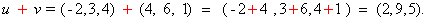### Subtracting vectors

Example 2:

Vectors v and u are given by their components as follows u = ( -2 , 3, 4 ) and v = ( 4 , 6, 1) . Find u - v.

Solution: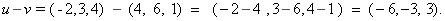### Multiplication by scalars:

Example 3:

Vector u are given by their components as follows u = ( -2 , 3, 4). Find 7u.

Solution: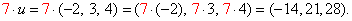### Vector magnitude

Example 4:

Vector u are given by their components as follows u = ( -2 , 3, 4). Find the magnitude of the vector u.

Note: The magnitude of vector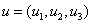is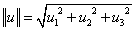.

Solution: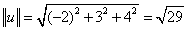.